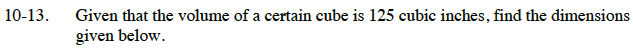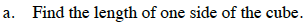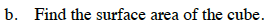### Home > CC3 > Chapter Ch10 > Lesson 10.1.1 > Problem10-13

10-13.
1. Given that the volume of a certain cube is 125 cubic inches, find the dimensions given below. Homework Help ✎

1. Find the length of one side of the cube.

2. Find the surface area of the cube.s3 = 125
s = ?Find the area of one face of the cube.

Multiply the area by the number of faces on the cube.

150 square inches